# Acids, Bases And Salts

##### Class 9th Science & Technology MHB Solution
Exercises
1. Identify the odd one out and justify. A. Chloride, nitrate, hydride, ammonium B. Hydrogen…
2. 50ml water is added to 50ml solution of copper sulphate. Write down the changes that will…
3. Two drops of the indicator phenolphthalein were added to 10ml solution of sodium…
4. Two or three filings of copper were added to 10ml dilute nitric acid and stirred. Write…
5. A litmus paper was dropped into 2ml dilute HCl. Then 2ml concentrated NaOH was added to it…
6. Magnesium oxide was added to dilute HCl and magnesium oxide was a added to dilute NaOH.…
7. Zinc oxide was added to dilute HCl and zinc oxide was added to dilute NaOH. Write down the…
8. Dilute HCl was added to limestone. Write down the changes that will be seen in each…
9. Pieces of blue vitriol were heated in a test tube. On cooling, water was added to it.…
10. Dilute H2SO4 was taken in an electrolytic cell and electric current was passed through it.…
11. Classify the following oxides into three types and name the types. CaO, MgO, CO2, SO3,…
12. Formation of sodium chloride from sodium and chlorine. Explain by drawing a figure of the…
13. Formation of a magnesium chloride from magnesium and chlorine. Explain by drawing a figure…
14. Show the dissociation of the following compounds on dissolving in water, with the help of…
15. 7.3g HCl in 100ml solution Write down the concentration of each of the following solutions…
16. 2g NaOH in 50ml solution Write down the concentration of each of the following solutions…
17. 3g CH3COOH in 100ml solution Write down the concentration of each of the following…
18. 4.9g H2SO4 in 200ml solution Write down the concentration of each of the following…
19. Obtain a sample of rainwater. Add to it a few drops of universal indicator. Meausre its…
20. Classify the acids according to their basicity and give one example of each type. Answer…
21. What is meant by neutralization? Give two examples from everyday life of the…
22. Explain what is meant by electrolysis of water. Write the electrode reactions and explain…
23. Give reason for the following. A. Hydronium ions are always in the form H3O+ B. Buttermilk…
24. Write the chemical equations for the following activities. A. NaOH solution was added to…
25. Acids and bases State the differences.
26. Cation and anion State the differences.
27. Negative electrode and positive electrode. State the differences.…
28. Classify aqueous solutions of the following substances according to their pH into three…
29. Collect information about electroplating. Make a list of articles in day-to-day life,…

###### Exercises
Question 1.

Identify the odd one out and justify.

A. Chloride, nitrate, hydride, ammonium

B. Hydrogen chloride, sodium hydroxide, calcium oxide, ammonia

C. Acetic acid, carbonic acid, hydrochloric acid, nitric acid

D. Ammonium chloride, sodium chloride, potassium nitrate, sodium sulphate

E. Sodium nitrate, sodium carbonate, sodium sulphate, sodium chloride

F. Calcium oxide, magnesium oxide, zinc oxide, sodium oxide.

G. Crystalline blue vitriol, crystalline common salt, crystalline ferrous sulphate, crystalline sodium carbonate.

H. Sodium chloride, potassium hydroxide, acetic acid, sodium acetate.

A. The odd one(s) – ammonium

Explanation: Ammonium(NH4+) is the odd one out because it is the only positively charged ion (cation). While chloride (Cl-), nitrate (NO-) and hydride (H-) are the negatively charged ion (anion)

B. The odd one(s) – Hydrogen chloride

Explanation: Hydrogen chloride is odd one out because it is the only acid among the given compounds. While sodium hydroxide (NaOH), calcium oxide (CaO) and ammonia (NH3) are the bases.

C. The odd one(s) – Acetic acid

Explanation: Acetic acid is odd one out because it is the only organic acid. While carbonic acid, hydrochloric acid and nitric acid are inorganic acids.

D. The odd one(s) – Ammonium chloride

Explanation: Ammonium chloride is the odd one out out

among the given compounds because it is an acidic salt. While

sodium chloride, potassium nitrate, sodium sulphate are the

neutral salts.

Note:

Acidic salts are formed by the neutralization reaction between a strong acid and a weak base.

Neutral salts are formed by the neutralization of a strong acid and a strong base.

Salts are formed by the combination of acid and base. The reaction takes place is called neutralization reaction.

E. The odd one(s) – Sodium chloride

Explanation: sodium chloride is the odd one out because it the only neutral salt present.

F. The odd one(s) – ZnO

Explanation: ZnO is odd one out because it is the only amphoteric oxides among the given oxides. While CaO, MgO and Na2O are basic oxides.

G. The odd one(s) – Crystalline common salt

Explanation: Crystalline common salt is the odd one out

because it does not lose its crystallization on heating.

H. The odd one(s) – Potassium hydroxide

Explanation: Potassium hydroxide is odd one out because it is not salt. While all others are salts

Question 2.

Write down the changes that will be seen in each instance and explain the reason behind it.

50ml water is added to 50ml solution of copper sulphate.

The changes observed when 50ml water is added to 50ml solution of copper sulphate are:

i. A reversible reaction takes place.
ii. Colour of copper sulphate changes.

iii. Anhydrous copper sulphate changes to hydrated copper sulphate.
Explanation: The changes occur due to the following reaction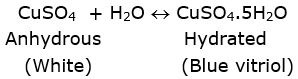The water of crystallization is taking place in the above reaction. Thus, the colour changes from white to blue

Question 3.

Write down the changes that will be seen in each instance and explain the reason behind it.

Two drops of the indicator phenolphthalein were added to 10ml solution of sodium hydroxide.

The change observed when two drops of the indicator phenolphthalein was added to 10ml solution of sodium hydroxide is:

The solution changes from colorless to dark pink in colour.

Explanation: Phenolphthalein is a basic indicator.

i. When it is mixed with any acid, it remains colourless.

ii. When mixed with any base, its colour changes to pink. NaOH is a base.

Thus, the solution becomes dark pink in colour.

Question 4.

Write down the changes that will be seen in each instance and explain the reason behind it.

Two or three filings of copper were added to 10ml dilute nitric acid and stirred.

The changes observed when two or three filings of copper were added to 10ml dilute nitric acid and stirred are:

i. The solution changes to bluish red.

ii. A red toxic gas is evolved.

iii. Copper is completely consumed.

Explanation: The changes occur due to the following reaction

Cu + 4HNO3→ Cu(NO3)2 + 2NO2 + 2H2O

In the above reaction, the copper metal is oxidized by nitric acid. The nitrogen dioxide (NO2) formed in the reaction is so dangerous and toxic.

Question 5.

Write down the changes that will be seen in each instance and explain the reason behind it.

A litmus paper was dropped into 2ml dilute HCl. Then 2ml concentrated NaOH was added to it and stirred.

The changes observed when a litmus paper was dropped into 2ml dilute HCl. Then 2ml concentrated NaOH was added to it and stirred are:

i. With HCl, the litmus paper turns red.

ii. With NaOH, the litmus paper turns blue.

Explanation: HCl is an acid and has pH lesser than 7, thus its

colour changes to red.

NaOH is a base and has pH greater than 7, thus its colour changes to blue.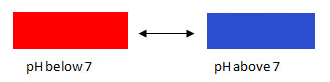Question 6.

Write down the changes that will be seen in each instance and explain the reason behind it.

Magnesium oxide was added to dilute HCl and magnesium oxide was a added to dilute NaOH.

The changes observe when magnesium oxide was added to dilute HCl and magnesium oxide was added to dilute NaOH are:

i. With HCl, magnesium oxide forms salt and water.

ii. With NaOH, no reaction takes place.

Explanation: When magnesium oxide being a basic oxide reacts with HCl (an acid), it forms a salt.

MgO + HCl → MgCl2 + H2O

The above reaction is an example of neutralization reaction.

On the other hand, when magnesium oxide being a basic oxide reacts with NaOH (a base), no reaction takes place. Because to carry out a neutralization reaction, there must be a combination of acid and base. But here both are bases, that’s why no reaction takes place.

Question 7.

Write down the changes that will be seen in each instance and explain the reason behind it.

Zinc oxide was added to dilute HCl and zinc oxide was added to dilute NaOH.

The changes observe when zinc oxide was added to dilute HCl and zinc oxide was added to dilute NaOH are:

i. With HCl, zinc oxide forms salt and water which shows basic properties.

ii. With NaOH, zinc oxide forms salt and water which shows acidic properties.

Explanation: Zinc oxide is an amphoteric oxide which shows both the properties of an acid as well as a base.

When zinc oxide reacts with HCl (acid), shows basic properties:
ZnO + HCl → ZnCl2 + H2O (basic nature)

When zinc oxide reacts with NaOH (base), shows basic properties:
ZnO +NaOH → Na2ZnO2 + H2O (acidic nature)

Question 8.

Write down the changes that will be seen in each instance and explain the reason behind it.

Dilute HCl was added to limestone.

The changes observe when dilute HCl was added to limestone are:

i. Effervescence of carbon dioxide gas is formed.

ii. Bubbles are also produced.

Explanation: When dilute HCl was added to limestone (CaCO3), effervescence of carbon dioxide is formed due to the following reaction:

CaCO3 + 2HCl → CaCl2 + H2O + CO2

Question 9.

Write down the changes that will be seen in each instance and explain the reason behind it.

Pieces of blue vitriol were heated in a test tube. On cooling, water was added to it.

The changes observe when pieces of blue vitriol were heated in a test tube and on cooling, water was added to it are:

i. On heating, the crystalline structure of blue vitriol broke down to form a colorless powder.

ii. On adding water, the color changes back to blue color.

Explanation: When blue vitriol (hydrated CuSO4) is heated, it becomes anhydrous and colorless (white).

CuSO4.5H2O → CuSO4 + 5H2O

(blue) (white)

Now, when water was added, it regained its blue colour and becomes hydrated again.

CuSO4 + H2O → CuSO4.5H2O

(white) (hydrated)

Question 10.

Write down the changes that will be seen in each instance and explain the reason behind it.

Dilute H2SO4 was taken in an electrolytic cell and electric current was passed through it.

The changes observe when dilute H2SO4 was taken in an electrolytic cell and electric current was passed through it are:

i. Conduction of electricity becomes better.

ii. SO42- remains in the solution.

The acid makes the electrolytic cell a better conductor. The reaction takes place is:

H2SO4→ 2H+ + SO42-

Question 11.

Classify the following oxides into three types and name the types.

CaO, MgO, CO2, SO3, Na2O, ZnO, AI2O3, Fe2O3

There are three types of oxides:

i. Acidic oxides: Acidic oxides are the oxides of non-metals. When these oxides are combined with water, they form acid and when combined with base, form salts.

CO2 and SO3 are the acidic oxides

ii. Basic oxides: Basic oxides are the oxides of metals. They combined with water to form hydroxides and when combined with acid, they form salts.

CaO, MgO and Na2O are the basic oxides

iii. Amphoteric oxides: These are metallic oxides and exhibit the property of both acid and base.

When react with an acid , they form salt and water, this shows the basic properties. When react with a base, they form salt and water, this shows the acidic properties.

ZnO, Al2O3 and Fe2O3 are the amphoteric oxides

Question 12.

Explain by drawing a figure of the electronic configuration.

Formation of sodium chloride from sodium and chlorine.

A.The atomic number of sodium(Na) = 11

The electronic configuration of 11Na = 2,8,1

The atomic number of chlorine(Cl) = 17

The electronic configuration of 17Cl = 2,8,7

Na transfer its one electron to Cl, so that it can achieve noble

gas configuration (2,8) whereas Cl accepts one electron from Na so that it can achieve noble gas configuration (2,8,8) as shown below: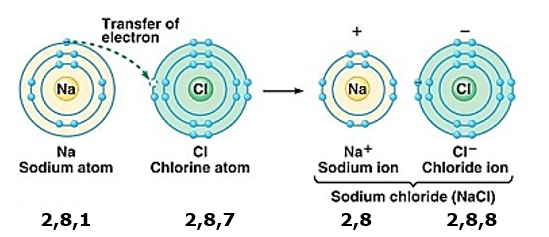Question 13.

Explain by drawing a figure of the electronic configuration.

Formation of a magnesium chloride from magnesium and chlorine.

The atomic number of magnesium(Mg) = 12

The electronic configuration of 12Mg = 2,8,2

The atomic number of chlorine(Cl) = 17

The electronic configuration of 17Cl = 2,8,7

Mg transfer its two electrons to each Cl, so that it can

achieve noble gas configuration (2,8) whereas each Cl accepts one electron from Mg so that both can achieve noble gas

configuration as shown below: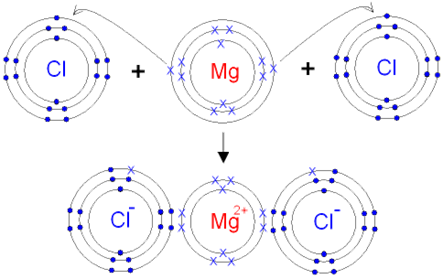Question 14.

Show the dissociation of the following compounds on dissolving in water, with the help of chemical equation and write whether the proportion of dissociation is small or large.

Hydrochloric acid, Sodium chloride, Potassium hydroxide, Ammonia, Acetic acid, Magnesium chloride, Copper sulphate.

1. Dissociation of hydrochloric acid in water

HCl → H+ + Cl-

The proportion of dissociation is large as 100% of the HCl dissociates into ions.

2. Dissociation of sodium chloride in water

NaCl → Na+ + Cl-

The proportion of dissociation is large as it completely dissolves in water.

3. Dissociation of potassium hydroxide in water

KOH → K+ + OH-

The proportion of dissociation is large as it completely dissolves in water.

4. Dissociation of ammonia in water

NH3 + H2O → NH4+ + OH-

The proportion of dissociation is large as it completely dissolves in water sue to formation of hydrogen bonding.

5. Dissociation of acetic acid in water

CH3COOH → CH3COO- + H+

The proportion of dissociation is small.

6. Dissociation of magnesium chloride in water

MgCl2→ Mg2+ + Cl-

The proportion of dissociation is large as magnesium chloride is highly soluble in water.

7. Dissociation of copper sulphate in water

CuSO4→ Cu2+ + SO42-

The proportion of dissociation is medium.

Question 15.

Write down the concentration of each of the following solutions in g/L and mol/L.

7.3g HCl in 100ml solution

Given:

Weight in grams= 7.3g

Volume in liters = 100 mL = 0.1L (1mL= 1/1000L)

Molecular mass of HCl = 1+ 35.4 = 36.4 g/mol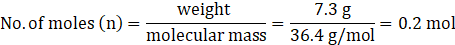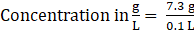Concentration in g/L = 73g/L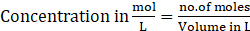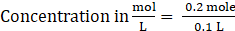Concentration in mol/L = 2 mol/L

Thus, concentration in g/L is 73g/L and concentration in mol/L is 2mol/L.

Question 16.

Write down the concentration of each of the following solutions in g/L and mol/L.

2g NaOH in 50ml solution

Given:

Weight in grams= 2g

Volume in liters = 50 mL = 0.05L (1mL= 1/1000L)

Molecular mass of NaOH = 23+16+1 = 40 g/mol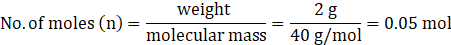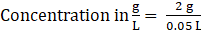Concentration in g/L = 40g/L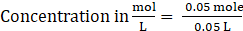Concentration in mol/L = 1 mol/L

Thus, concentration in g/L is 40g/L and concentration in mol/L is 1mol/L.

Question 17.

Write down the concentration of each of the following solutions in g/L and mol/L.

3g CH3COOH in 100ml solution

Given:

Weight in g= 3g

Volume in L = 100 mL = 0.1L (1mL= 1/1000L)

Molecular mass of CH3COOH = 12+3×1+12+16+16+1

= 60 g/mol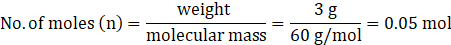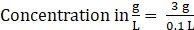Concentration in g/L = 30g/L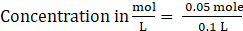Concentration in mol/L = 0.5 mol/L

Thus, concentration in g/L is 30g/L and concentration in mol/L is 0.5mol/L.

Question 18.

Write down the concentration of each of the following solutions in g/L and mol/L.

4.9g H2SO4 in 200ml solution

Given:

Weight in grams= 4.9g

Volume in liters = 200 mL = 0.2L (1mL= 1/1000L)

Molecular mass of H2SO4 = 2×1+32+4×16 = 98 g/mol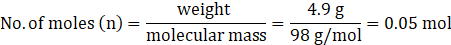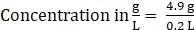Concentration in g/L = 24.5g/L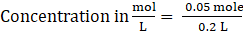Concentration in mol/L = 0.25 mol/L

Thus, concentration in g/L is 24.5g/L and concentration in mol/L is 0.25mol/L.

Question 19.

Obtain a sample of rainwater. Add to it a few drops of universal indicator. Meausre its pH. Describe the nature of the sample of rainwater and explain the effect if it has on the living world.

If the pH of rainwater is less than 7, it means its nature is acidic.

If the pH of rainwater is above 7, it means its nature is basic.

If the pH of rainwater is 7, it means its nature is neutral.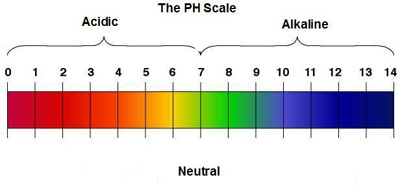Natural rainwater has a pH around 5.6 and is slightly acidic. If value of pH is below pH, then it is considered as “acid rain”. Acid rain particularly damages:

i. Lakes, rivers, streams

ii. Forests

iii. Plants and animals

iv. Human beings (especially damages the skin)

Question 20.

Classify the acids according to their basicity and give one example of each type.

Basicity of acids: The number of H+ ions obtainable by the dissociation of one molecule of an acid is called its basicity.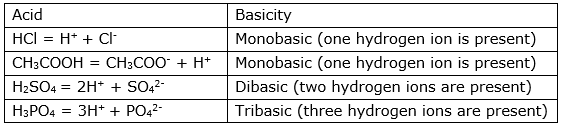Question 21.

What is meant by neutralization? Give two examples from everyday life of the neutralization reaction.

In the neutralization reaction, an acid reacts with a base to form a salt and water.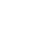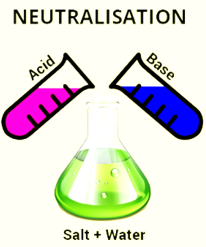Everyday examples:

i. Toothpastes help in neutralizing the effect of acid because they are alkaline in nature and neutralize the effect of acid present in our mouth.

ii. Use of baking soda in cooking.

iii. To reduce acidity in the stomach, we take milk of magnesia (Mg(OH)2).

Question 22.

Explain what is meant by electrolysis of water. Write the electrode reactions and explain them.

Electrolysis of water: It is defined as the process of decomposition of water into hydrogen and oxygen gas by the passage of electricity through its aqueous solution (water).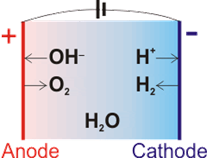i. At the cathode (negative electrode), reduction takes place and evolves hydrogen gas:

Reduction reaction: 2H+ + 2e-→ H2

ii. At the anode (positive electrode), osidation takes place and evolves oxygen gas:

Oxidation reaction: 2H2O → O2 + 4H+ + 4e-

iii. Overall cell reaction: 2H2O → 2H2 + O2

Question 23.

Give reason for the following.

A. Hydronium ions are always in the form H3O+

B. Buttermilk spoils if kept in a copper or brass container.

A. Dissociation of water molecule

H2O ↔ H+ + OH-

As soon as the H+ ions are formed, they make bond with water molecules in the solution to form hydronium ion(H3O+). This is because hydrogen cannot exist independently(alone) in the aqueous solutions, and hence take the form of the hydronium ion.

H2O + H+↔ H3O+

Water has ability to attract H+ because it is a polar molecule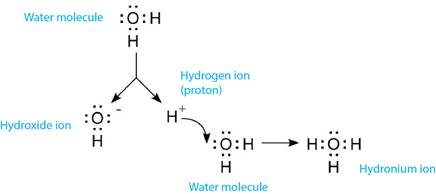B. Buttermilk spoils if kept in a copper or brass container because

i. Butter milk contains lactic acid.

ii. The lactic acid present in the buttermilk reacts completely with copper or brass container.

iii. Due to the chemical reaction taking place, the buttermilk becomes unfit for consumption and spoils the buttermilk.

Question 24.

Write the chemical equations for the following activities.

A. NaOH solution was added to HCl solution.

B. Zinc dust was added to dilute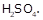C. Dilute nitric acid was added to calcium oxide.

D. Carbon dioxide gas was passed through KOH solution.

E. Dilute HCl was poured on baking soda.

A. When NaOH solution was added to HCl solution, it forms sodium chloride and liberated hydrogen gas.

HCl + NaOH → NaCl + H2

B. When zinc dust was added to dilute H2SO4, it forms zinc sulphate and liberated hydrogen gas.

H2SO4 + Zn → ZnSO4 + H2

C. When dilute nitric acid was added to calcium oxide, it forms calcium nitrate and water.

2HNO3 + CaO → Ca(NO3)2 + H2O

D. When carbon dioxide gas was passed through KOH solution; it was completely absorbed by KOH.

CO2 + KOH → KHCO3

E. When dilute HCl was poured on baking soda (NaHCO3), it forms a salt NaCl, water and releases bubbles of carbon dioxide.

NaHCO3 + HCl → NaCl + H2O+ CO2

The above reaction is an example of neutralization reaction.

Question 25.

State the differences.

Acids and bases

Difference between acids and bases: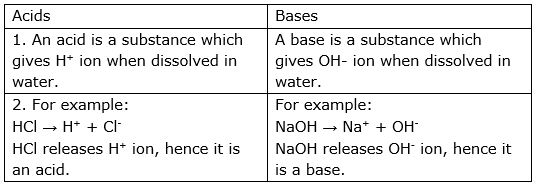Question 26.

State the differences.

Cation and anion

Difference between cation and anion: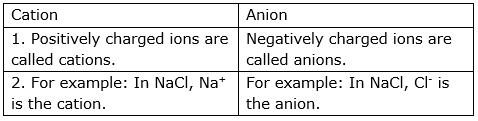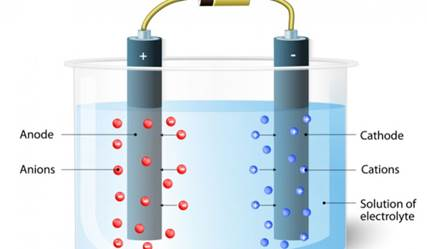Representation of cation and anions

Question 27.

State the differences.

Negative electrode and positive electrode.

Difference between Negative electrode and positive electrode: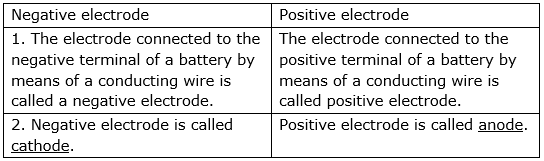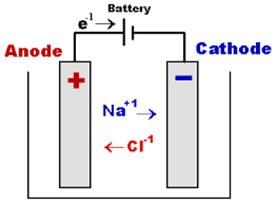Representation of anode and cathode

Question 28.

Classify aqueous solutions of the following substances according to their pH into three groups : 7, more than 7, less than 7.

Common salt, sodium acetate, hydrochloric acid, carbon dioxide, potassium bromide, calcium hydoxide, ammonium chloride, vinegar, sodium carbonate, ammonia, sulphur dioxide.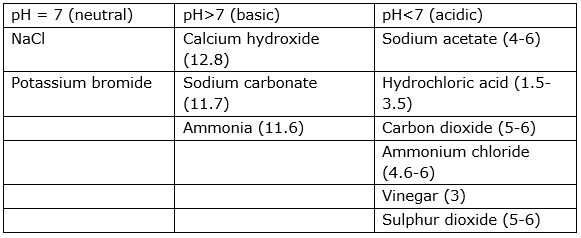Question 29.

Collect information about electroplating. Make a list of articles in day-to-day life, where this technique is used.

Electroplating: In this process, electric current is passed through electrolyte.

i. Two electrodes are dipped in the solution.

ii. Connecting with a circuit and a battery.

iii. The electrodes and electrolyte are made from chosen elements.

iv. On passing electric current, splitting of electrolyte takes place and some of its parts deposits to one of the electrode at the top

v. As a result, one of the electrode becomes electroplated.

Basically, the electroplating is the coating one metals with another metal.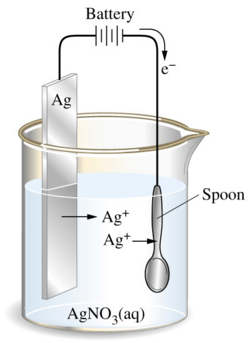Electroplating of silver onto spoon

List of articles in day to day life where electroplating is used:

i. Gold and silver coating is done in ornaments.

ii. Iron is coated with tin to make fresh containers.

iii. In machine parts, zinc coating is done.

iv. In cars, nickel coating is done.

v. In handle of cycles and wheels, chromium coating is done to give an extra shine.

## PDF FILE TO YOUR EMAIL IMMEDIATELY PURCHASE NOTES & PAPER SOLUTION. @ Rs. 50/- each (GST extra)

SUBJECTS

HINDI ENTIRE PAPER SOLUTION

MARATHI PAPER SOLUTION
SSC MATHS I PAPER SOLUTION
SSC MATHS II PAPER SOLUTION
SSC SCIENCE I PAPER SOLUTION
SSC SCIENCE II PAPER SOLUTION
SSC ENGLISH PAPER SOLUTION
SSC & HSC ENGLISH WRITING SKILL
HSC ACCOUNTS NOTES
HSC OCM NOTES
HSC ECONOMICS NOTES
HSC SECRETARIAL PRACTICE NOTES

2019 Board Paper Solution

HSC ENGLISH SET A 2019 21st February, 2019

HSC ENGLISH SET B 2019 21st February, 2019

HSC ENGLISH SET C 2019 21st February, 2019

HSC ENGLISH SET D 2019 21st February, 2019

SECRETARIAL PRACTICE (S.P) 2019 25th February, 2019

HSC XII PHYSICS 2019 25th February, 2019

CHEMISTRY XII HSC SOLUTION 27th, February, 2019

OCM PAPER SOLUTION 2019 27th, February, 2019

HSC MATHS PAPER SOLUTION COMMERCE, 2nd March, 2019

HSC MATHS PAPER SOLUTION SCIENCE 2nd, March, 2019

SSC ENGLISH STD 10 5TH MARCH, 2019.

HSC XII ACCOUNTS 2019 6th March, 2019

HSC XII BIOLOGY 2019 6TH March, 2019

HSC XII ECONOMICS 9Th March 2019

SSC Maths I March 2019 Solution 10th Standard11th, March, 2019

SSC MATHS II MARCH 2019 SOLUTION 10TH STD.13th March, 2019

SSC SCIENCE I MARCH 2019 SOLUTION 10TH STD. 15th March, 2019.

SSC SCIENCE II MARCH 2019 SOLUTION 10TH STD. 18th March, 2019.

SSC SOCIAL SCIENCE I MARCH 2019 SOLUTION20th March, 2019

SSC SOCIAL SCIENCE II MARCH 2019 SOLUTION, 22nd March, 2019

XII CBSE - BOARD - MARCH - 2019 ENGLISH - QP + SOLUTIONS, 2nd March, 2019

HSC Maharashtra Board Papers 2020

(Std 12th English Medium)

HSC ECONOMICS MARCH 2020

HSC OCM MARCH 2020

HSC ACCOUNTS MARCH 2020

HSC S.P. MARCH 2020

HSC ENGLISH MARCH 2020

HSC HINDI MARCH 2020

HSC MARATHI MARCH 2020

HSC MATHS MARCH 2020

SSC Maharashtra Board Papers 2020

(Std 10th English Medium)

English MARCH 2020

HindI MARCH 2020

Hindi (Composite) MARCH 2020

Marathi MARCH 2020

Mathematics (Paper 1) MARCH 2020

Mathematics (Paper 2) MARCH 2020

Sanskrit MARCH 2020

Important-formula

THANKS